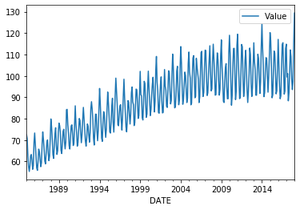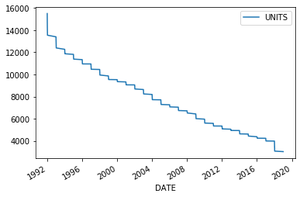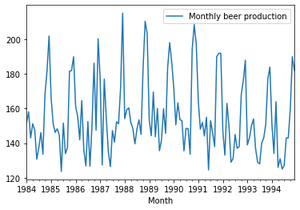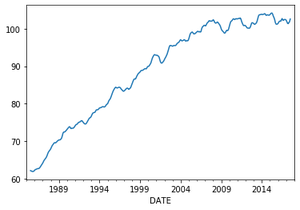# What is a trend in time series?

• Last Updated : 24 Jan, 2021

Time series data is a sequence of data points that measure some variable over ordered period of time. It is the fastest-growing category of databases as it is widely used in a variety of industries to understand and forecast data patterns. So while preparing this time series data for modeling it’s important to check for time series components or patterns.  One of these components is Trend.

Trend is a pattern in data that shows the movement of a series to relatively higher or lower values over a long period of time. In other words, a trend is observed when there is an increasing or decreasing slope in the time series. Trend usually happens for some time and then disappears, it does not repeat. For example, some new song comes, it goes trending for a while, and then disappears. There is fairly any chance that it would be trending again.

Attention geek! Strengthen your foundations with the Python Programming Foundation Course and learn the basics.

To begin with, your interview preparations Enhance your Data Structures concepts with the Python DS Course. And to begin with your Machine Learning Journey, join the Machine Learning - Basic Level Course

A trend could be :

• Uptrend: Time Series Analysis shows a general pattern that is upward then it is Uptrend.
• Downtrend: Time Series Analysis shows a pattern that is downward then it is Downtrend.
• Horizontal or Stationary trend: If no pattern observed then it is called a Horizontal or stationary trend.

You can find trends in data either by simply visualizing or by the decomposing dataset.

### Visualization

By simply plotting the dataset you can see the general trend in data

Approach :

• Import module
• Cast month column to date time object
• Set month as index
• Create plot

Note: In the examples given below the same code is used to show all three trends just the dataset used is different to reflect that particular trend.

Link for datasets : cick here

Example: Uptrend

## Python3

 `# importing the libraries``import` `pandas as pd``import` `matplotlib`` ` `# importing dataset``data ``=` `pd.read_csv(r``'C:\Users\admin\Downloads\Electric_Production.csv'``)`` ` `# casting Month column to datetime object``data[``'DATE'``] ``=` `pd.to_datetime(data[``'DATE'``])`` ` `# Setting Month as index``data ``=` `data.set_index(``'DATE'``)`` ` `# Creating the plot``data.plot()`

Output :Example: Downtrend

## Python3

 `import` `pandas as pd``import` `matplotlib`` ` `# importing dataset``data ``=` `pd.read_csv(r``'C:\Users\admin\Downloads\AlcoholSale.csv'``)`` ` `# casting Date column to datetime object``data[``'DATE'``] ``=` `pd.to_datetime(data[``'DATE'``])`` ` `# Setting Date column as index``data ``=` `data.set_index(``'DATE'``)`` ` `# Creating the plot``data.plot()`

Output :Example: Horizontal trend

## Python3

 `# importing the libraries``import` `pandas as pd``import` `matplotlib`` ` `# importing dataset``data ``=` `pd.read_csv(``    ``r``'C:\Users\admin\Downloads\monthly-beer-production-in-austr.csv'``)`` ` `# casting Month column to datetime object``data[``'Month'``] ``=` `pd.to_datetime(data[``'Month'``])`` ` `# Setting Month as index``data ``=` `data.set_index(``'Month'``)`` ` `# Creating the plot``data[``'1984'``:``'1994'``].plot()`

Output :Decomposition

To see the complexity behind linear visualization we can decompose the data. The function called seasonal_decompose within the statsmodels package can help us to decompose the data into its components/show patterns — trend, seasonality and residual components of time series. Here we are interested in trend component only so will acces it using seasonal_decompose().trend .

seasonal_decompose function uses moving averages method to estimate the trend.

Syntax :

Important parameters :

• x : array-like.  Time-Series.  If 2d, individual series are in columns. x must contain 2 complete cycles.
• model : {“additive”, “multiplicative”}, optional (Depends on nature on seasonal component)
• period(freq.) : int, optional . Must be use if x is not pandas object or index of  x does not have a frequency.

Returns : A object with seasonal, trend, and resid attributes.

Example :

## Python3

 `# importing function``from` `statsmodels.tsa.seasonal ``import` `seasonal_decompose`` ` `# creating trend object by assuming multiplicative model``output ``=` `seasonal_decompose(data, model``=``'multiplicative'``).trend`` ` `# creating plot``output.plot()`

Output :My Personal Notes arrow_drop_up